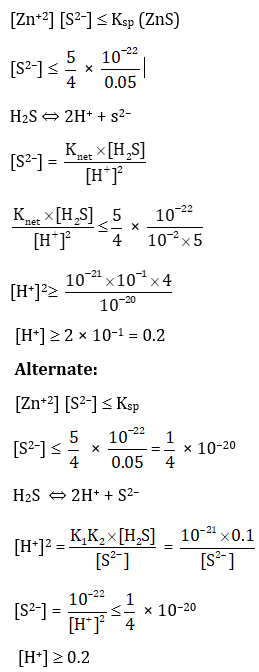Win up to 100% scholarship on Aakash BYJU'S JEE/NEET courses with ABNAT Win up to 100% scholarship on Aakash BYJU'S JEE/NEET courses with ABNAT

# JEE Advanced 2020 Chemistry Paper 2

Students can download solutions for JEE Advanced 2020 question paper 2 for Chemistry subject from this page. The questions have been solved in a detailed manner by subject experts at BYJU’S. Practising these questions will help the JEE aspirants to understand the overall exam pattern and prepare accordingly for upcoming exams. To score a higher rank in the JEE Advanced exams students are advised to practice previous years’ papers.
Paper 1 – Chemistry

Question 1: The 1st, 2nd, and the 3rd ionization enthalpies, 𝐼1, 𝐼2, and 𝐼3, of four atoms with atomic numbers n, 𝑛 + 1, 𝑛 + 2, and 𝑛 + 3, where 𝑛 < 10, are tabulated below. What is the value of n?

 Atomic Number Ionization Enthalpy (kJ/mol) I1 I2 I3 n 1681 3374 6050 n+1 2081 3952 6122 n+2 469 4562 6910 n+3 738 1451 7733

According to the tabulated data,

Element with Atomic number (n+2), should be an alkali metal

As we see, first ionization enthalpy (I1) is very less but second ionization enthalpy (I2) is very large.

Hence, atomic number can be = 11

That is, (n + 2) = 11

n = 9

Note: ‘n’ can’t be ‘1’.

Question 2: Consider the following compounds in the liquid form:

O2, HF, H2O, NH3, H2O2, CCl4, CHCl3, C6H6, C6H5Cl.

When a charged comb is brought near their flowing stream, how many of them show deflection as per the following figure?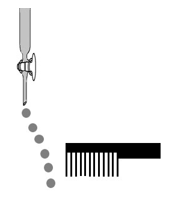Only polar molecules are deflected by charged comb.

Polar molecules: HF, H2O, NH3, H2O2, CHCl3, C6H5Cl

Non-polar molecules: O2, CCl4, Benzene

Question 3: In the chemical reaction between stoichiometric quantities of KMnO4 and KI in weakly basic solution, what is the number of moles of I2 released for 4 moles of KMnO4 consumed?

Chemical reaction of KMnO4 and KI in weakly basic solution is given as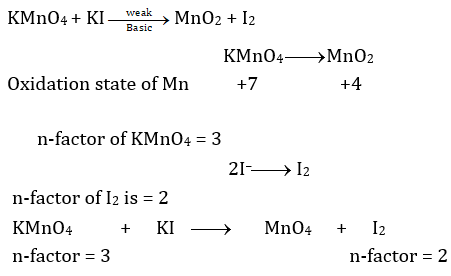Equivalents of KMnO4= Equivalents of I2

n-factor × Number of moles (n) = n-factor × Number of moles (n)

3 × moles of KMnO4 = 2 × moles of I2

3 × 4 = 2 × moles of I2

Moles of I2 = 6 moles

Question 4: An acidified solution of potassium chromate was layered with an equal volume of amyl alcohol. When it was shaken after the addition of 1 mL of 3% H2O2, a blue alcohol layer was obtained. The blue color is due to the formation of a chromium (VI) compound ‘X’. What is the number of oxygen atoms bonded to chromium through only single bonds in a molecule of X?

Blue colour compound of ‘Cr’ is CrO5.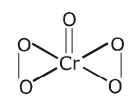Oxygen atoms bonded to chromium through only single bonds = 4

Question 5: The structure of a peptide is given below.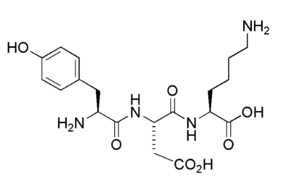If the absolute values of the net charge of the peptide at pH = 2, pH = 6, and pH = 11 are |𝑧1|, |𝑧2|, and |𝑧3|, respectively, then what is |𝑧1| + |𝑧2| + |𝑧3|?

(i) At pH = 2 (Highly acidic)

In highly acidic medium, the given tripeptide exists as cationic form.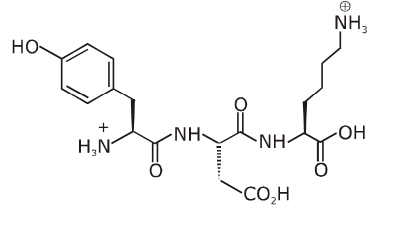Net charge +2

|Z1| = 2 at pH = 2

(ii)At pH = 6 (neutral solution)

In neutral medium, the given tripeptide exists as Zwitter ion.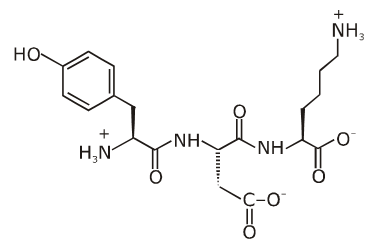net charge = 0

|Z2| = 0 at pH = 6

(iii)At pH = 11 (basic medium)

In basic medium the given tripeptide exists in anionic form.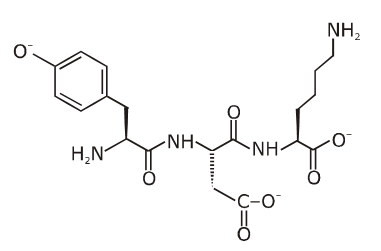Net charge = –3

|Z3| = 3

Therefore, |Z1| + |Z2| + |Z3| = 2 + 0 + 3 = 5

Question 6: An organic compound (C8H10O2) rotates plane-polarized light. It produces pink color with neutral FeCl3 solution. What is the total number of all the possible isomers for this compound?

DBE (Double bond equivalent) of C8H10O2 is

= Number of carbon atoms – (Number of monovalent atoms)/2 + 1

= 8 – 10/2 + 1 = 4

It gives pink colour with neutral FeCl3 solution. It means phenolic group should be present in the compound.

Note: C* represent chiral carbon. So it will have (d and l) optically active isomers.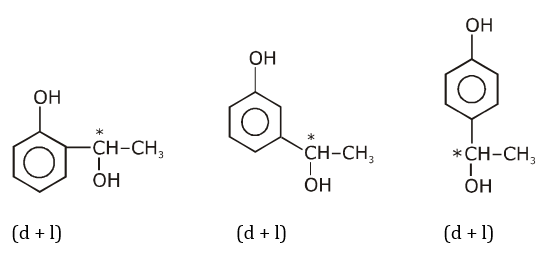Total optically active isomer = 6

Question 7: In an experiment, m grams of a compound X (gas/liquid/solid) taken in a container is loaded in a balance as shown in figure I below. In the presence of a magnetic field, the pan with X is either deflected upwards (figure II), or deflected downwards (figure III), depending on the compound X. Identify the correct statement(s).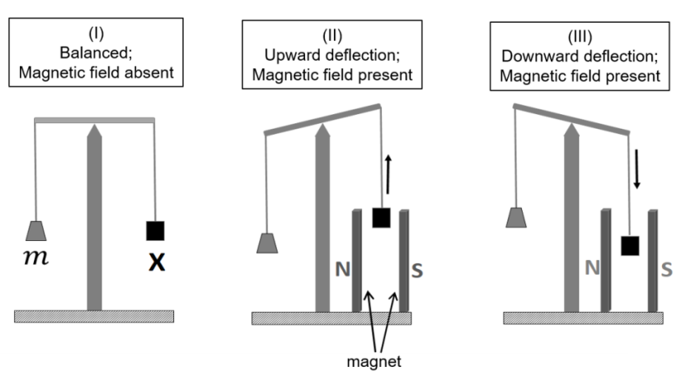a) If X is H2O(l), deflection of the panis upwards.
b) If X is K4[Fe(CN)6](𝑠), deflection of the panis upwards.
c) If X is O2 (𝑔), deflection of the panis downwards.
d) If X is C6H6(l), deflection of the panis downwards.

Paramagnetic substances are attracted by magnetic fields & diamagnetic substances are repelled by magnetic field.

O2 – is paramagnetic

H2O & C6H6(l) – are Diamagnetic

& K4[Fe(CN)6] is also Diamagnetic

a) x = H2O &rarr; Diamagnetic

b) x = K4[Fe(CN)6] &rarr; Diamagnetic

Here, Fe+2 strong field ligand.

&rarr; 3d6 &rArr; [t2g6, eg0]

c) x = O2 &rarr; Paramagnetic

Here, O2(g) is paramagnetic due to two unpaired electrons present in pi* (antibonding orbitals)

d) x = C6H6 (l) &rarr; diamagnetic (incorrect)

It is due to presence of 0 unpaired electrons.

Question 8: Which of the following plots is (are) correct for the given reaction?

([P]0 is the initial concentration of P)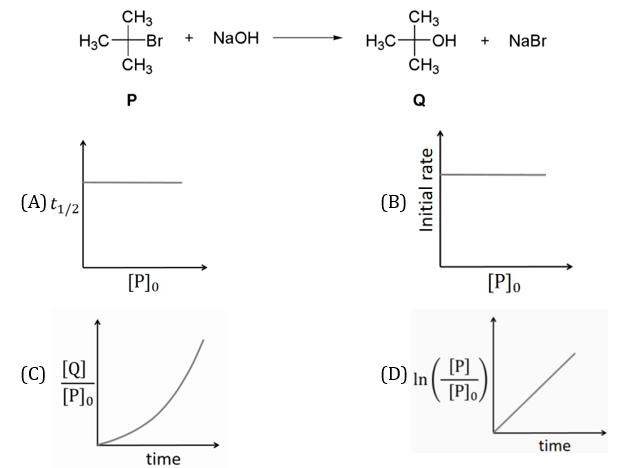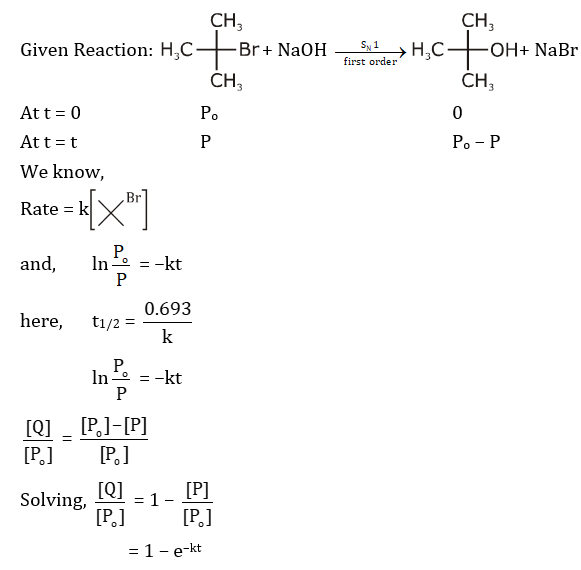As there is no inversion. Hence should be

SN1 &rarr; 1st order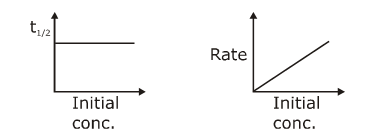c) x = a{1 – e–kt}

x/a = 1 – e–kt

x/a = Q/P0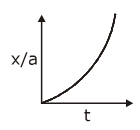Question 9: Which among the following statement(s) is(are) true for the extraction of aluminium from bauxite?

a) Hydrated Al2O3 precipitates, when CO2 is bubbled through a solution of sodium aluminate.
b) Addition of Na3AlF6 lowers the melting point of alumina.
c) CO2 is evolved at the anode during electrolysis.
d) The cathode is a steel vessel with a lining of carbon.

Refer topic metallurgy

Refer topic metallurgy

a)Extraction of aluminium (Hall’s process and Hall Heroult’s electrolytic cell):

The process involved in extraction of aluminium is Hall Heroult’s process.

During process, Al2O3 is obtained as precipitate.

When CO3 is bubbled through a solution of sodium aluminate.

The reaction is given as:

2Na[Al(OH)4](aq.) + CO2 &rarr; Na2CO3 + H2O + 2Al(OH)3 (&darr;) or Al2O3.2H2O (ppt)

Refer topic metallurgy

b) Electrolytic reduction of pure alumina takes place in steel box with lining of carbon (cathode) with cryolite (Na3AlF6) and fluorspar (CaF2) which lowers the melting point and increases the conductivity of electrolyte.

Refer topic metallurgy

c) Electrolysis process in Hall’s process:

Graphite rods acts as anode:

At cathode:

Al+3 + 3e &rarr; Al

At anode: The oxygen liberated at anode reacts with the carbon of anode to produce CO and CO2.

C + O2– &rarr; CO + 2e

C + 2O2– &rarr; CO2 + 4e

Refer topic metallurgy

d)Here the cathode is a steel vessel with a lining of carbon.

Question 10: Choose the correct statement(s) among the following.

a) SnCl2.2H2O is a reducing agent.
b) SnO2 reacts with KOH to form K2[Sn(OH)6].
c) A solution of PbCl2 in HCl contains Pb2+and Clions.
d) The reaction of Pb3O4 with hot dilute nitric acid to give PbO2 is a redox reaction.

a)SnCl2.2H2O is a reducing agent since Sn2+ tends to convert into Sn4+

SnO2 + KOH &rarr; K2SnO3 + H2O

or

Amphoteric K2[Sn(OH)6]

b)First group cations (Pb2+) form insoluble chloride with HCl that is PbCl2 however it is slightly soluble in water and therefore lead +2 ion is never completely precipitated on adding hydrochloric acid in test sample of Pb2+, rest of the Pb2+ ions are quantitatively precipitated with H2S in acidic medium.

So that we can say that filtrate of first group contain solution of PbCl2 in HCl which contains Pb2+and Cl.

However, in the presence of conc. HCl or excess HCl it can produce H2[PbCl4].

So, we can conclude A, B or A,B,C should be answers

PbCl2 + HCl → H2 [PbCl4]

Pb3O4 + HNO3 → PbO2 + Pb(NO3)2 + H2O

or

2PbO.PbO2 (Non redox reaction)

Question 11: Consider the following four compounds I, II, III, and IV.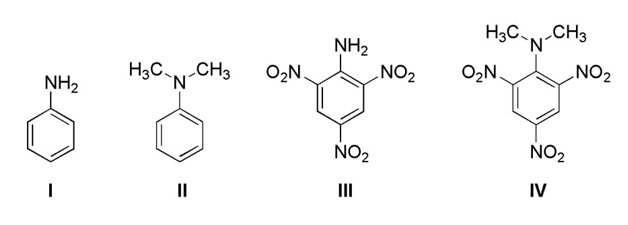Choose the correct statement(s).

a) The order of basicity is II >I >III >IV.
b) The magnitude of pKb difference between I and II is more than that between III and IV.
c) Resonance effect is more in III than in IV.
d) Steric effect makes compound IV more basic than III.

a)Correct basic strength order of given compound is

(IV) > (II) > (I) > (III)

b) Compound IV is a stronger base than III due to SIR effect, which basic strength difference between I & II is very less.

c) In compound IV due to SIR (steric inhibition due to resonance) effect both –NO2 and N(CH3)2 group will be out of plane hence resonance effect in compound IV is less.

Question 12: Consider the following transformations of a compound P.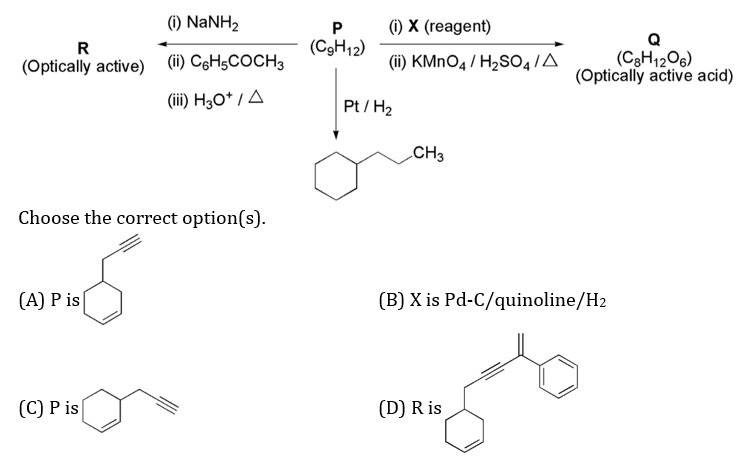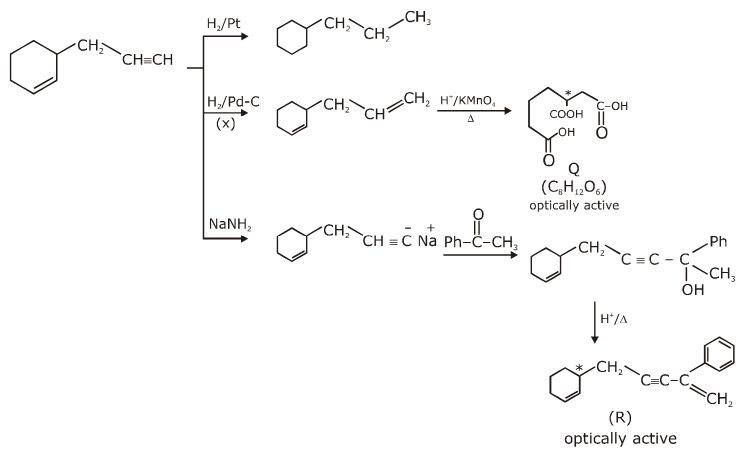• NaNH2 acts as a base for the double elimination of geminal or vicinal dihalides to give alkynes.

• Pd-C / quinoline + H2 &rArr; a lindlar’s catalyst that reduce only alkynes not alkenes.

Question 13: A solution of 0.1 M weak base (B) is titrated with 0.1 M of a strong acid (HA). The variation of pH of the solution with the volume of HA added is shown in the figure below. What is the p𝐾b of the base? The neutralization reaction is given by

B + HA &rarr; BH+ + A.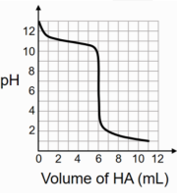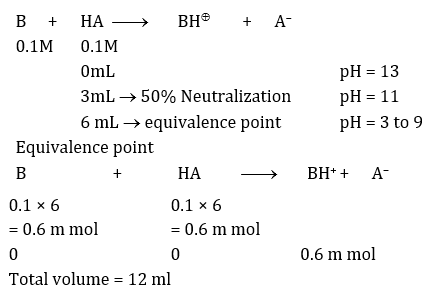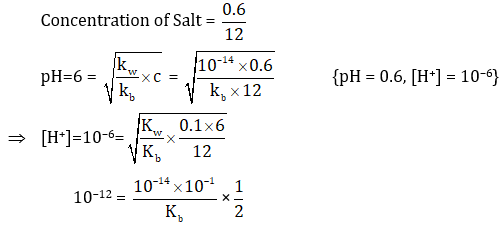kb = 5 × 10–4

pkb = –logkb = –log(5 × 10–4) = –log5 + 4log10

pkb= 4 – 0.7

pkb= 3.3

Question 14: Liquids A and B form ideal solution for all compositions of A and B at 25℃. Two such solutions with 0.25 and 0.50 mole fractions of A have the total vapor pressures of 0.3 and 0.4 bar, respectively. What is the vapor pressure of pure liquid B in bar?

PTotal = 0.3, where xA =

xA + xB = 1

&rArr; xB = 0.75 =

&rArr; 0.3= + …(1)

PTotal = 0.4 where xA=, xB =

&rArr; 0.4= + .…(2)

Apply eq.(1) × 2 – eq.(2), we get

= 0.2 bar

Question 15: The figure below is the plot of potential energy versus internuclear distance (𝑑) of H2 molecule in the electronic ground state. What is the value of the net potential energy 𝐸0 (as indicated in the figure) in kJ mol−1, for 𝑑 = 𝑑0 at which the electron-electron repulsion and the nucleus-nucleus repulsion energies are absent? As reference, the potential energy of H atom is taken as zero when its electron and the nucleus are infinitely far apart.

Use Avogadro constant as 6.023 × 1023 mol−1.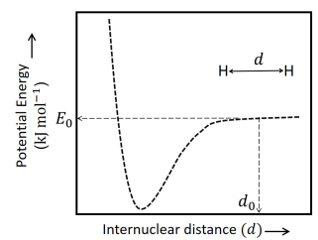P. E of 2 H-atoms

Total eng = P.E./2

Potential Energy = 2 Total Energy

E = -13.6 x z2/n2 ev/atom

= –2 x 13.6 x z2/n2 ev/atom + (–2 x 13.6 x z2/n2) ev/ atom

= – 2x 2 x 13.6 x 1 x ev/atom

= – 4 x 13.6 x 1.6 x 10–19 J/atom × 6.023 × 1023 atom/mole

= – 4 × 13.6 × 1.6 × 6.023×104 J/mole

= – 5242.42 KJ/mol

Question 16: Consider the reaction sequence from P to Q shown below. The overall yield of the major product Q from P is 75%. What is the amount in grams of Q obtained from 9.3 mL of P? (Use density of P = 1.00 g mL−1; Molar mass of C = 12.0, H =1.0, O =16.0 and N = 14.0 g mol−1)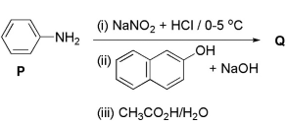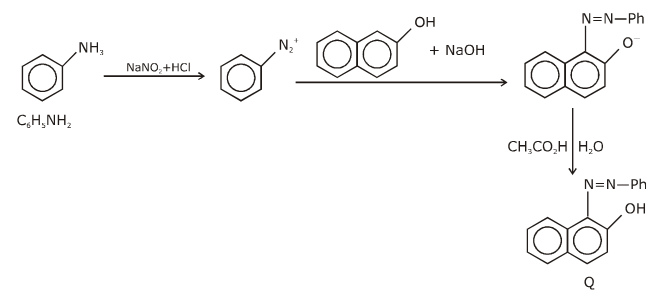Molecular weight of aniline (C6H5NH2) = 77 + 14 + 2 = 93

Density of P = 1 gm ml–1

d = m/v

or m = dv

Mass of P = 9.3 × 1 = 9.3 g

9.3 ml of P = 9.3 gm

P = of P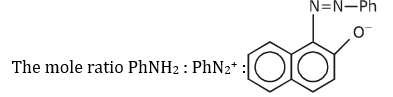= 1:1:1

So,the mole of Q formed will be 0.1 mole and extent of reaction is 100% but if it is 75% yield.

Then amount of Q = 0.1 x 75/100 = 0.075 mol

The molecular formula of Q = C16H12ON2

So, M. wt. of Q = 16 x 12 + 12 x 1 + 16 + 2 x 14

= 192 + 12 + 16 + 28

= 248 gm / mol

So, amount of Q = 248 x 0.075= 18.6 gm

Question 17: Tin is obtained from cassiterite by reduction with coke. Use the data given below to determine the minimum temperature (in K) at which the reduction of cassiterite by coke would take place.

At 298 K: ∆𝑓𝐻0(SnO2(𝑠)) = −581.0 kJ mol−1, ∆𝑓𝐻0(CO2(g)) = −394.0 kJ mol−1,

𝑆0(SnO2(s)) = 56.0J K−1mol−1, 𝑆0(Sn(s)) = 52.0 J K−1mol−1,

𝑆0(C(𝑠)) = 6.0J K−1mol−1, 𝑆0(CO2(g)) = 210.0 J K−1mol−1.

Assume that the enthalpies and the entropies are temperature independent.

SnO2 (s) + C(s) &rarr; CO2 + Sn

&Delta;H = (&Delta;f H)P – (&Delta;f H)R

= – 394 + 581

= + 187 KJ/mol

&Delta;S = (&Delta; S)P – (&Delta; S)R

= 210 + 52 – 56 – 6

= 200 J/k mol

&Delta; G = 187 × 1000 – 200 × T

T = [187×1000]/200 = 935 K

Question 18: An acidified solution of 0.05 MZn2+ is saturated with 0.1 M H2S. What is the minimum molar concentration (M) of H+ required to prevent the precipitation of ZnS?

Use 𝐾sp(ZnS) = 1.25 x 10-22 and overall dissociation constant of H2S,

KNET = 𝐾1𝐾2 = 1 × 10-21.